"Multiplication Kids: Math Game" App Analytics: ASO Keyword Monitoring | ASOTools

# "Multiplication Kids: Math Game" App Analytics: ASO Keyword Monitoring | ASOTools

Are you doing Multiplication Kids: Math Game app analytics for better app store ASO strategies? Whether Multiplication Kids: Math Game in app store keep on top? What keyword tactics Multiplication Kids: Math Game take to rank well in app store? Are app store keywords like "multiplication games", "multiply", "multiplication for kids" matter in Multiplication Kids: Math Game ranking?

Free ASO tools for Google Play & App Store, ASOTools will reveal the app store keywords secrets of Multiplication Kids: Math Game.

## App Analytics: Multiplication Kids: Math Game

Multiplication Kids: Math Game-Multiplication Games for Kids, published by RV AppStudios LLC in app store, released on 2019-04-04, and last updated 2021-03-04. It belongs to all category, and all category is ranking no rank in app store. Currently, Multiplication Kids: Math Game has 599 ratings, the average rating is 4. Additionally, it had - downloads and - revenue last month in app store worldwide, and the daily active users is -.

More Multiplication Kids: Math Game info and other app analytics can be found freely in ASOTool.

## ASO Keyword Monitoring: Multiplication Kids: Math Game

### 1.Multiplication Kids: Math Game Top 10 App Store Keywords

 Keyword Search Volume KD Related Apps Multiplication Kids: Math Game's Rank multiplication games 42 5289 28 194 1 multiply 17 4605 18 185 1 multiplication for kids 7 1059 16 190 1 multiplication kids: math game - - 28 190 1 timetable games 3 - 33 223 1 the flash games free mode - - 13 3 1 multiplication kids 1 - 32 186 1 learn multiplication to kids - - 14 193 1 mutiplication games 4 - 29 196 1 multiplication games free - - 20 230 1

App store keywords matter for ASO. If your app is similar to Multiplication Kids: Math Game, when you optimize app store keyword ranking, pay attention to the metrics above. ASOTools will show you all of them for free.

Obviously, Multiplication Kids: Math Game is ranking for "multiplication games", "multiply", "multiplication for kids", "multiplication kids: math game", "timetable games", "the flash games free mode", "multiplication kids", "learn multiplication to kids", "mutiplication games", and "multiplication games free" these top 10 keywords in app store.

Also, you'll find Multiplication Kids: Math Game's position in app store search result. Multiplication Kids: Math Game is ranking 1 for multiplication games, ranking 1 for multiply, ranking 1 for multiplication for kids, and ranking 1 for multiplication kids: math game. For more Multiplication Kids: Math Game keyword monitoring, please visit asotools.io.

### 2. Top 5 Keyword-Related Apps& App Analytics

• multiplication games  Related Apps & App Analytics
 Apps Average Star Ratings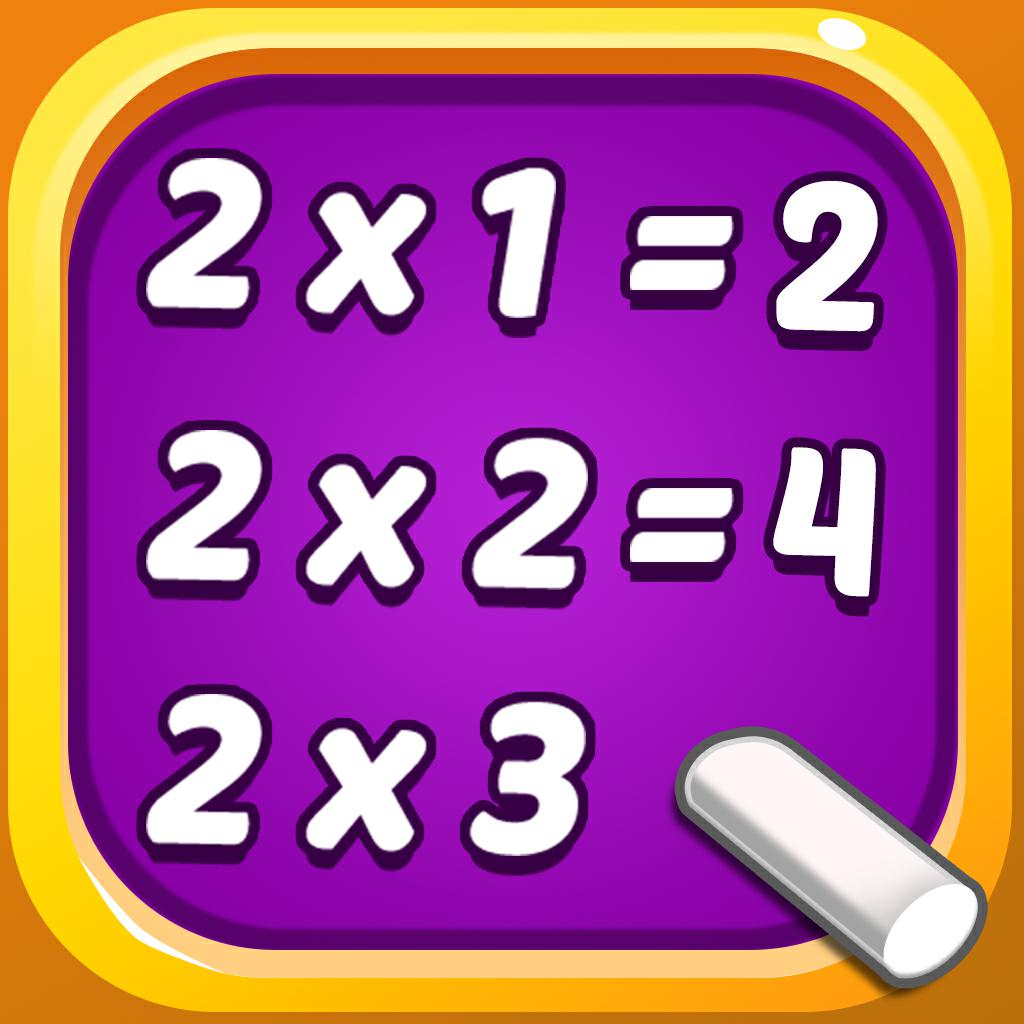Multiplication Kids: Math Game-Multiplication Games for Kids 4 599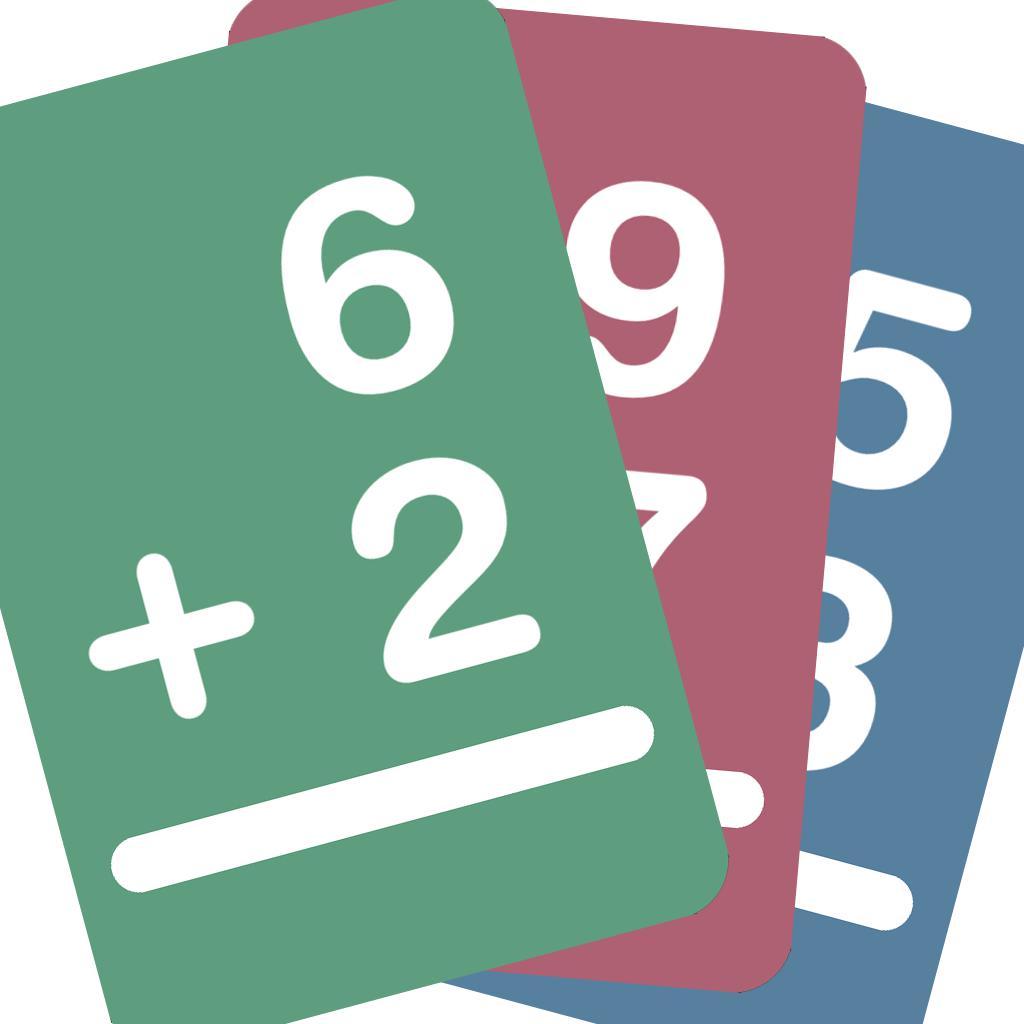Big Math Flash Cards-Add Subtract Multiply Divide 4 16884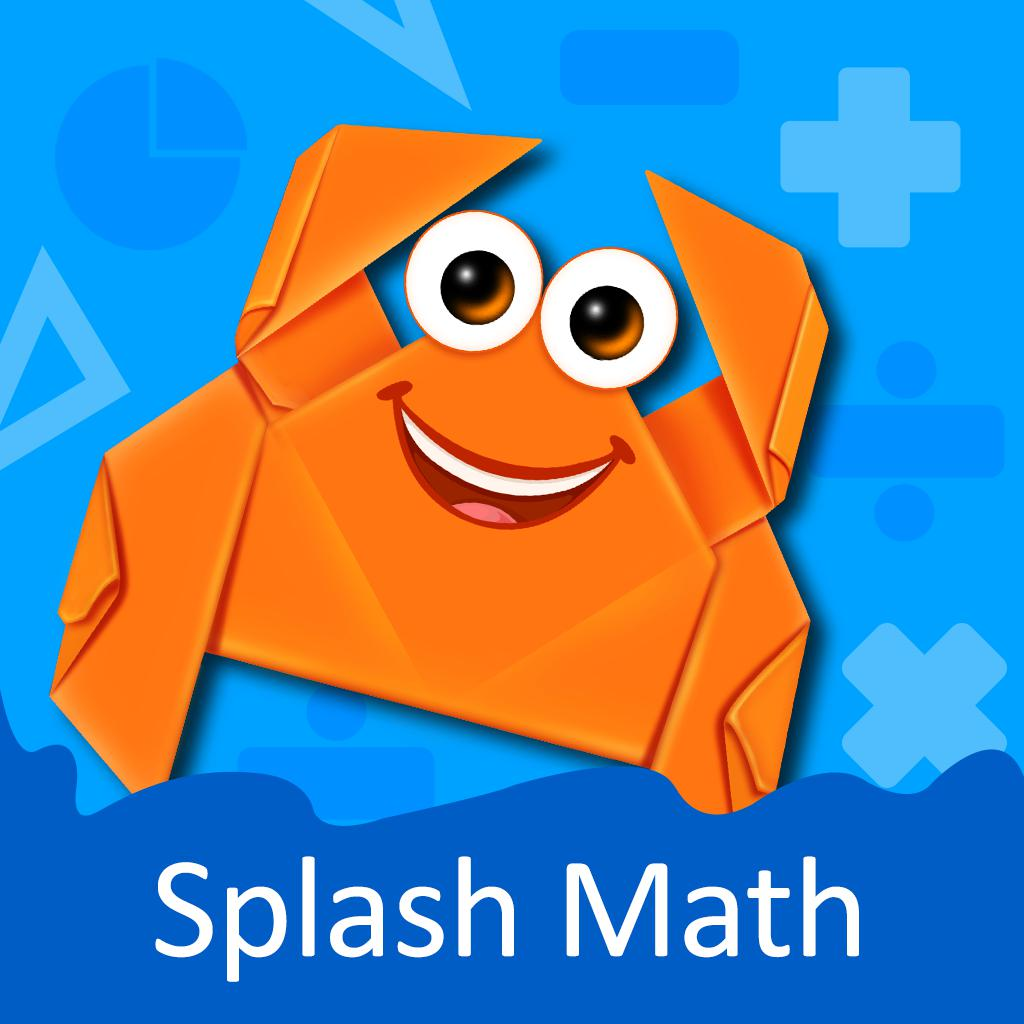3rd Grade Math Games for Kids-Cool app for 7 to 9 year olds 4 4991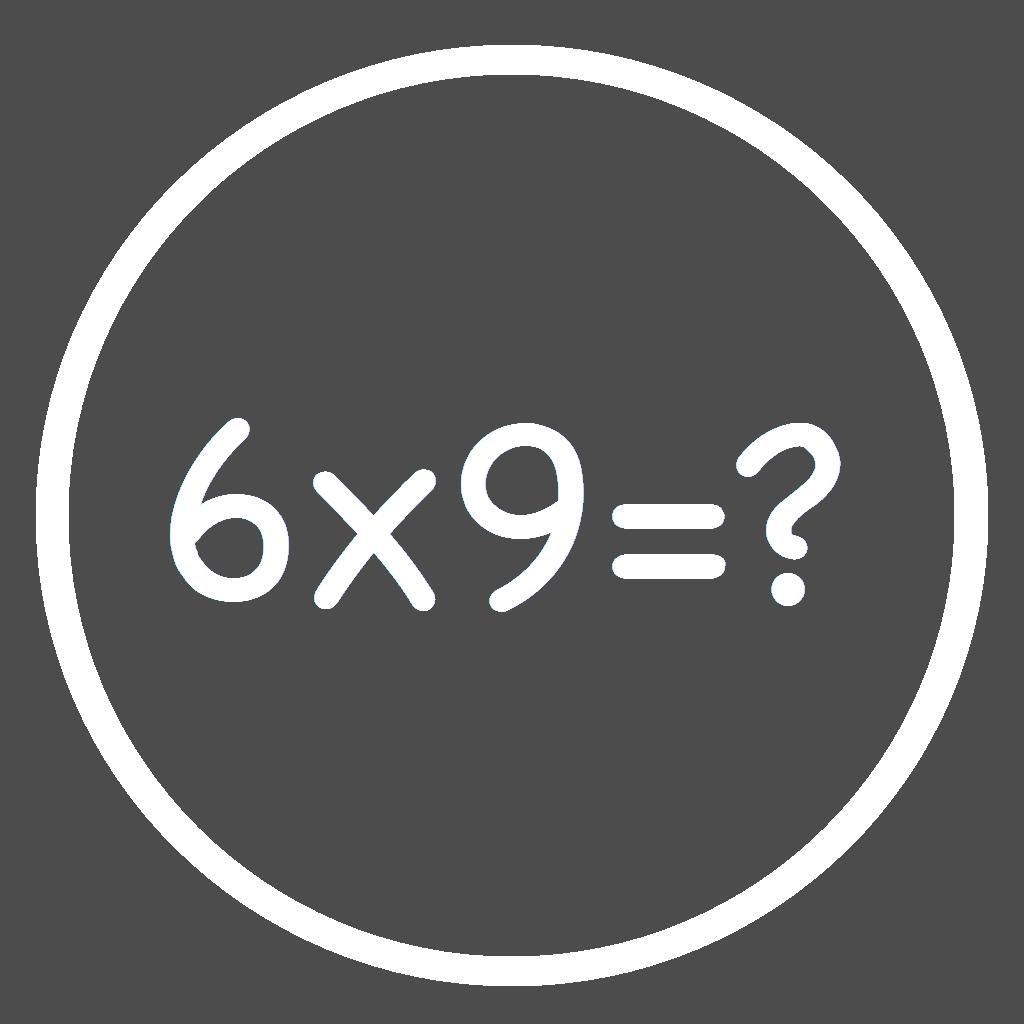Cool Times Tables Flash Cards-Multiplication with fun colors 4 8925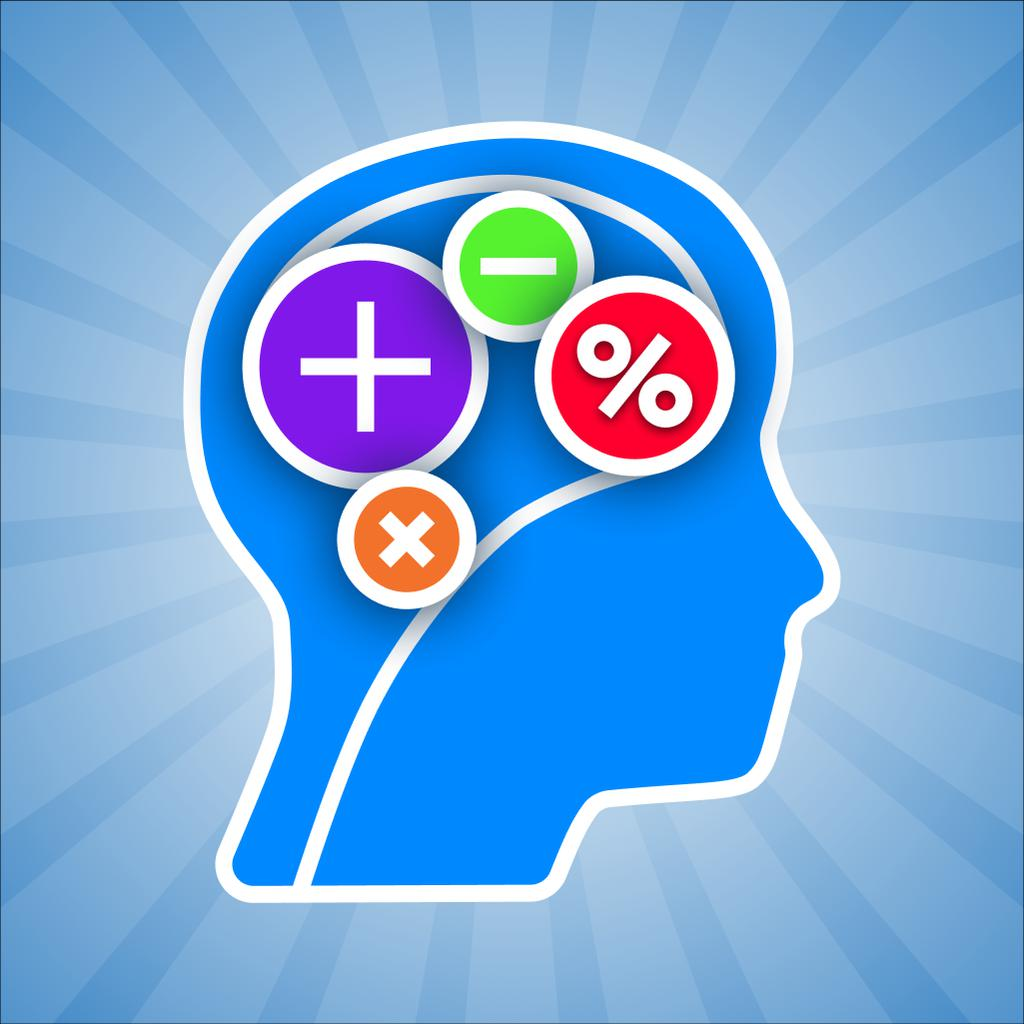Math Brain Booster Games-Play & Excel at Mental Math 4 17923

"multiplication games" has 194 apps. Top 5 are Multiplication Kids: Math Game, Big Math Flash Cards, 3rd Grade Math Games for Kids, Cool Times Tables Flash Cards, and Math Brain Booster Games.

• multiply  Related Apps & App Analytics
 Apps Average Star RatingsMultiplication Kids: Math Game-Multiplication Games for Kids 4 599Multiply: Disciples Making Disciples 5 13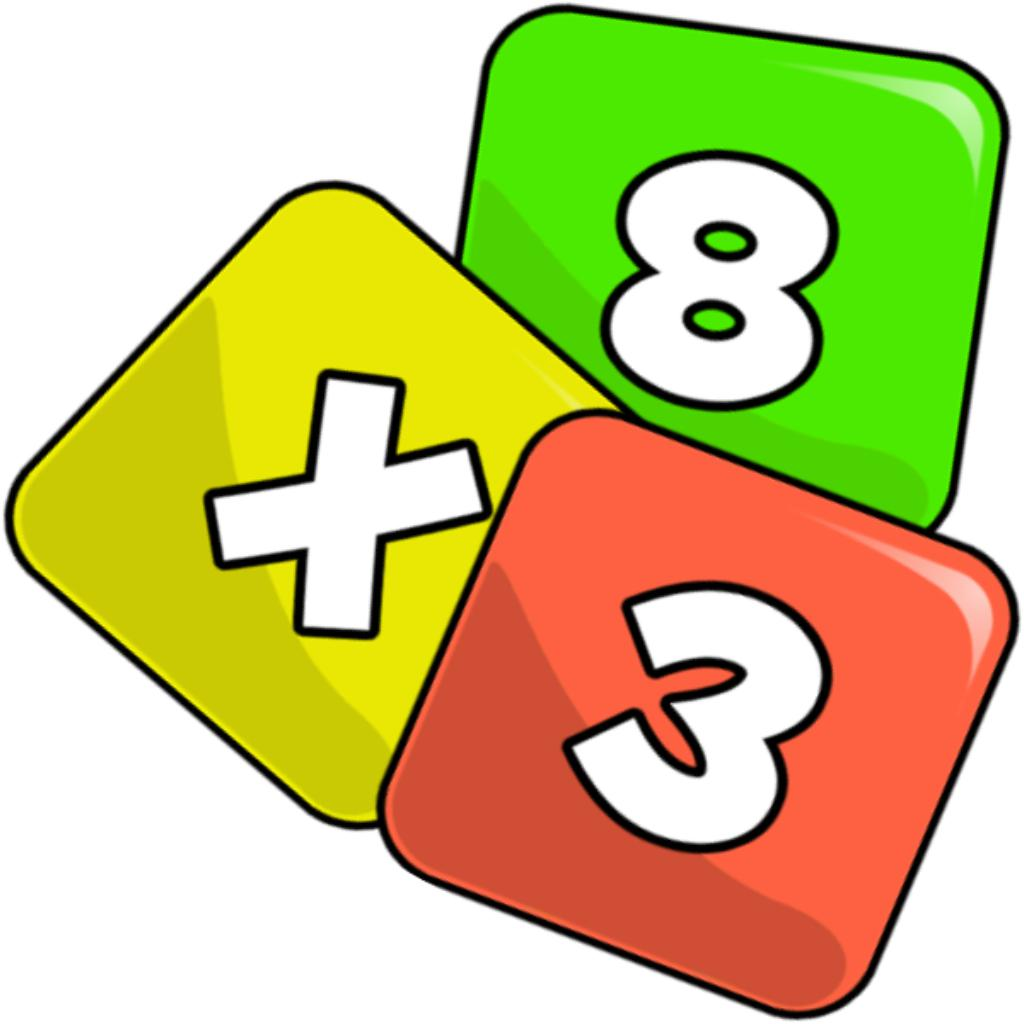Multiplication Tables. 4 369Multiplication: Times Tables-Multiply Math Fun Flash Cards 5 214Big Math Flash Cards-Add Subtract Multiply Divide 4 16884

"multiply" has 185 related apps in total. Top 5 are Multiplication Kids: Math Game, Multiply: Disciples Making Disciples, Multiplication Tables., Multiplication: Times Tables, and Big Math Flash Cards.

• multiplication for kids  Related Apps & App Analytics
 Apps Average Star RatingsMultiplication Kids: Math Game-Multiplication Games for Kids 4 599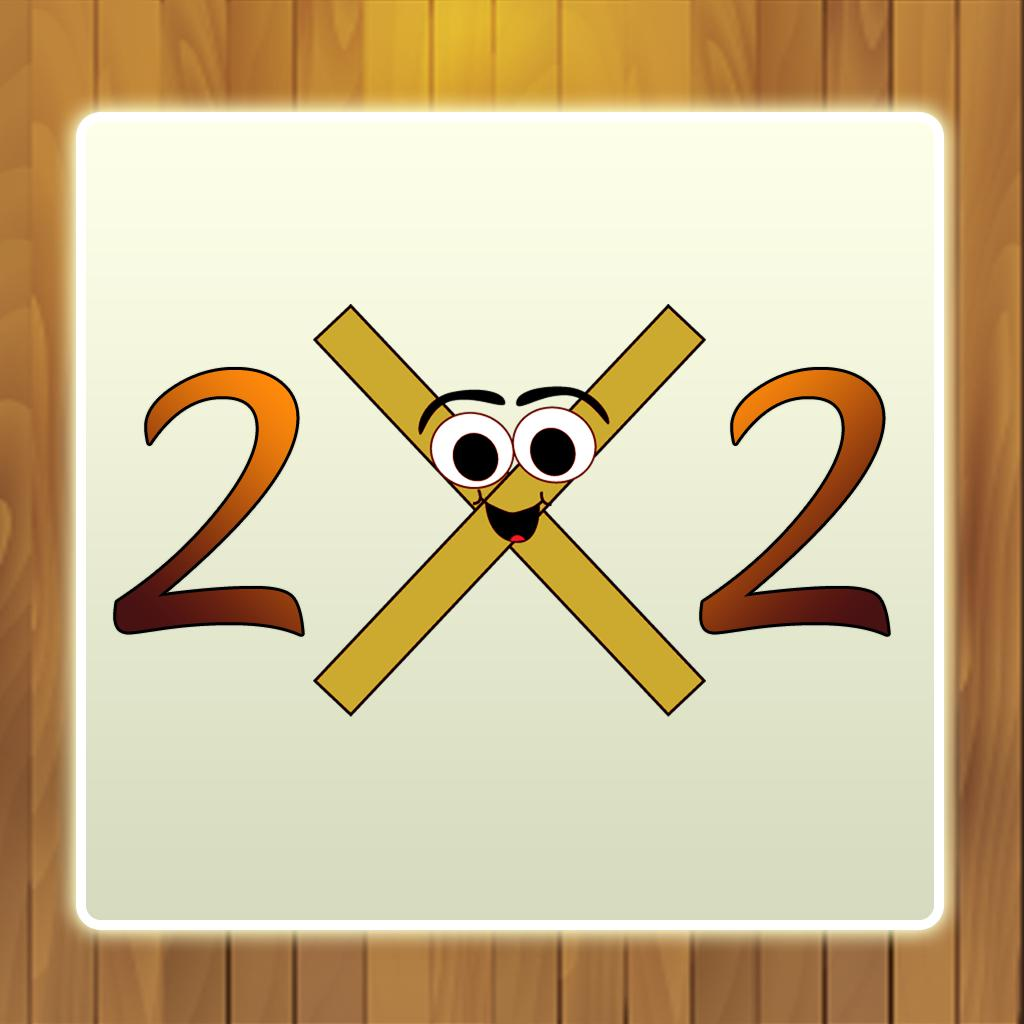Learn Multiplication to kids 3 5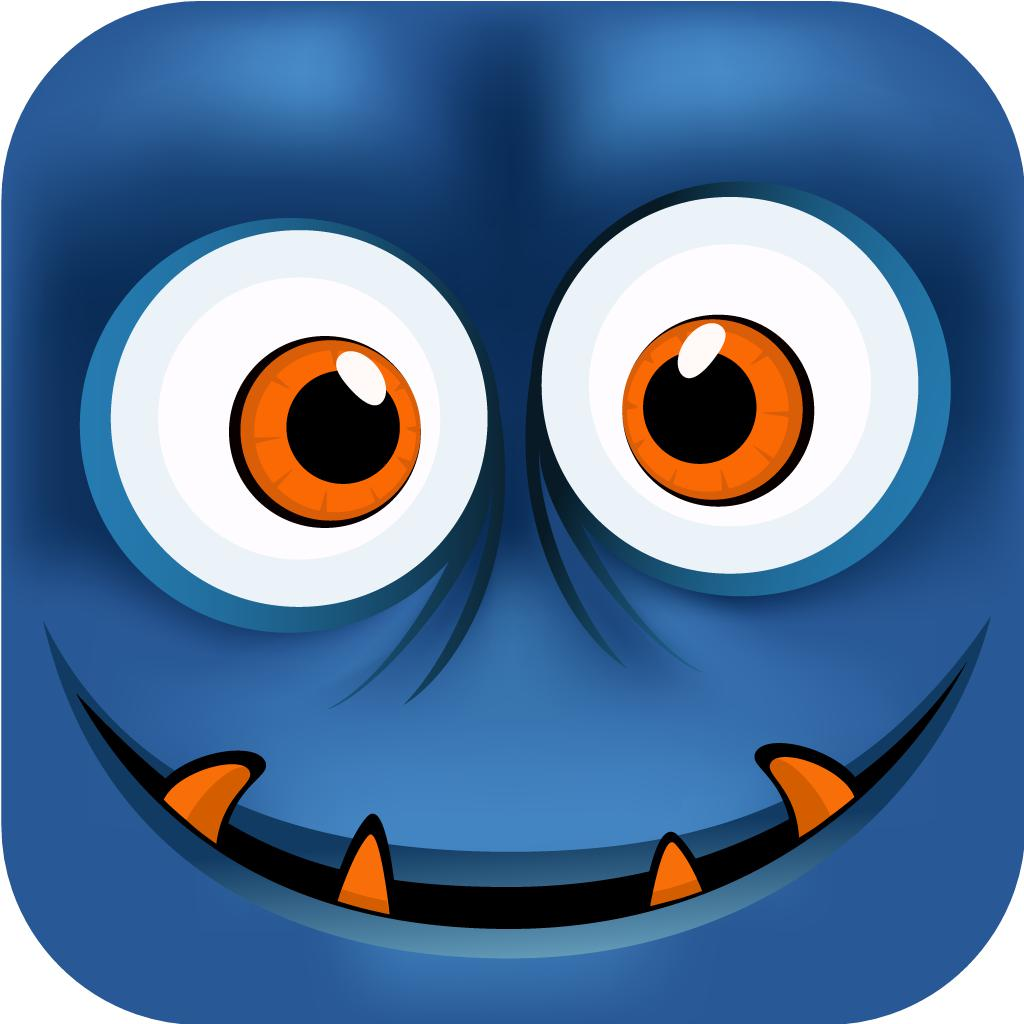Monster Math : Kids Fun Games-Facts Fluency for Grades K-5 4 5947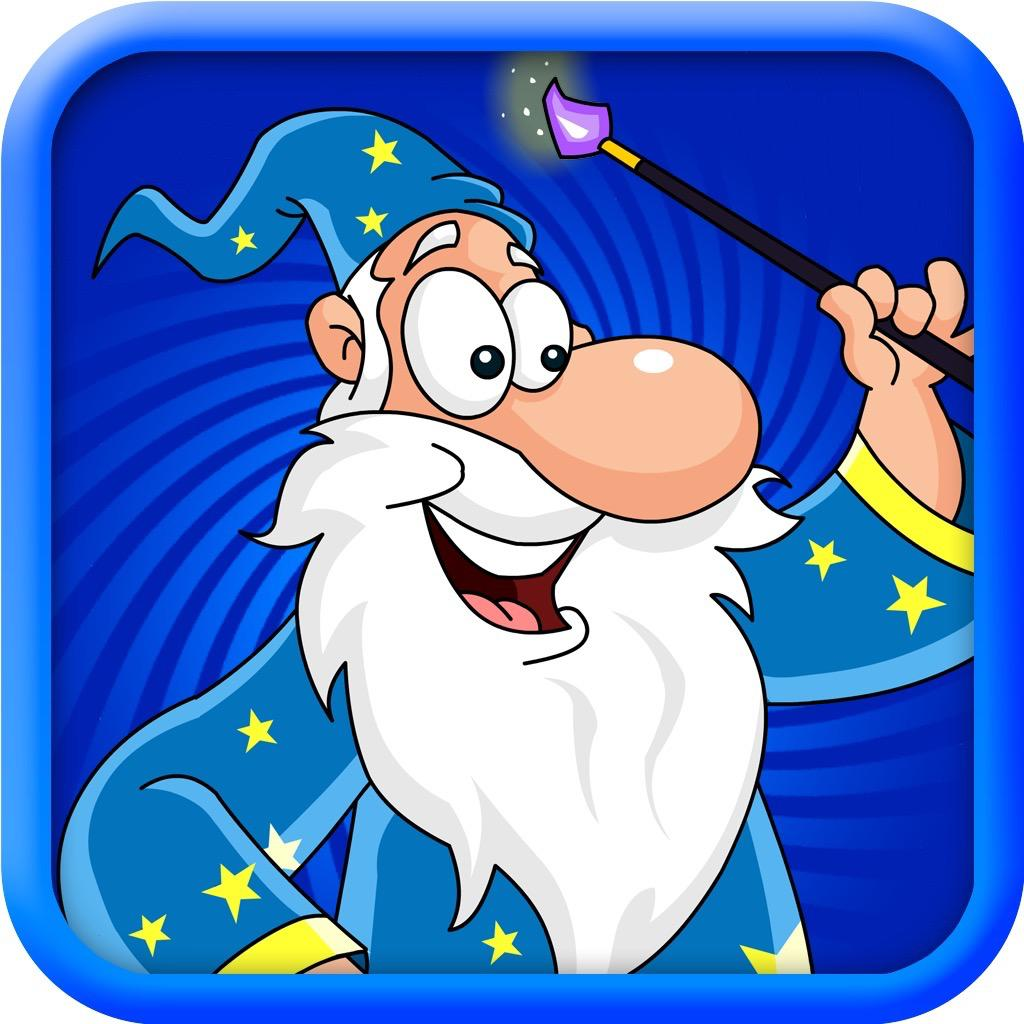Multiplication For Kids-Times Tables Chart & Games 3 7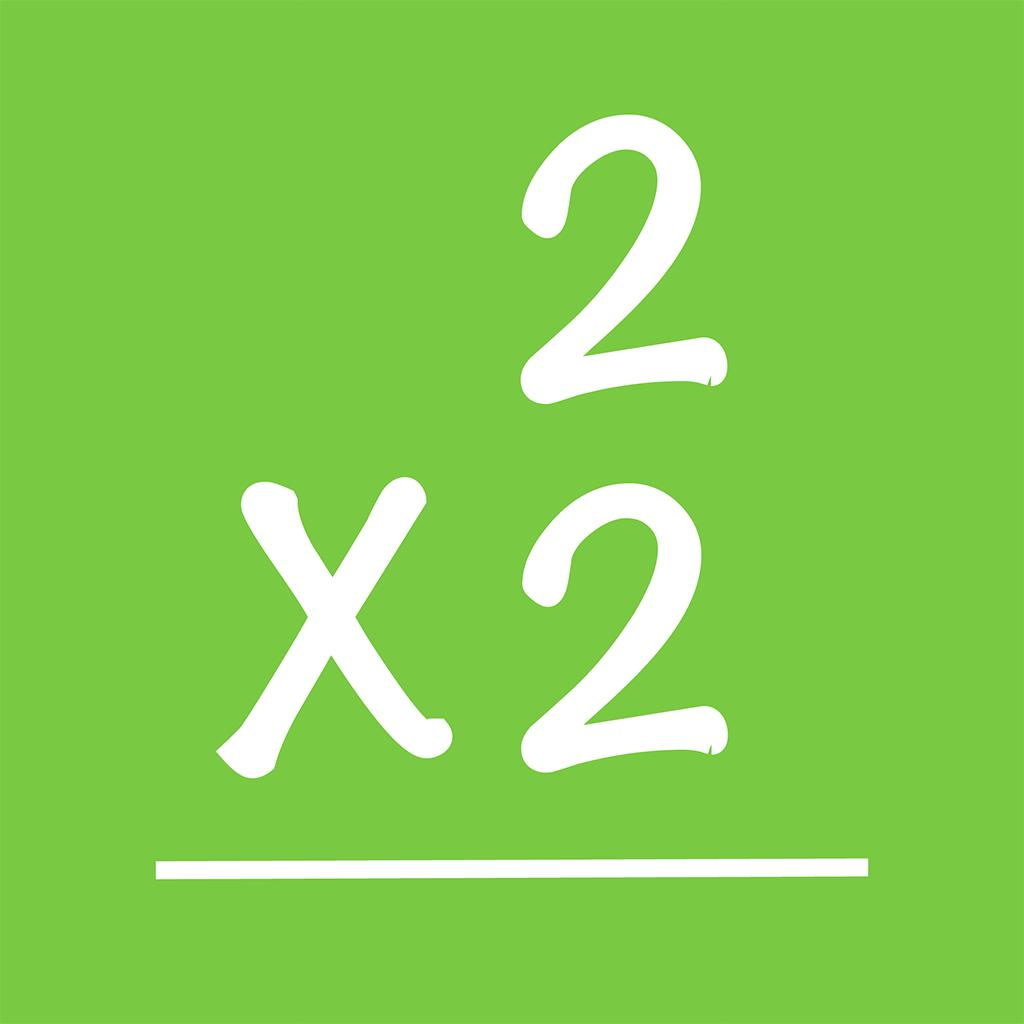Times Tables - Flash Quiz-Multiplication Flash Cards 4 4455

"multiplication for kids" has 190 related apps in app store. Top 5 are Multiplication Kids: Math Game, Learn Multiplication to kids, Monster Math : Kids Fun Games, Multiplication For Kids, and Times Tables - Flash Quiz.

• multiplication kids: math game  Related Apps & App Analytics
 Apps Average Star RatingsMultiplication Kids: Math Game-Multiplication Games for Kids 4 599Monster Math : Kids Fun Games-Facts Fluency for Grades K-5 4 5947Math Brain Booster Games-Play & Excel at Mental Math 4 17923Cool Times Tables Flash Cards-Multiplication with fun colors 4 8925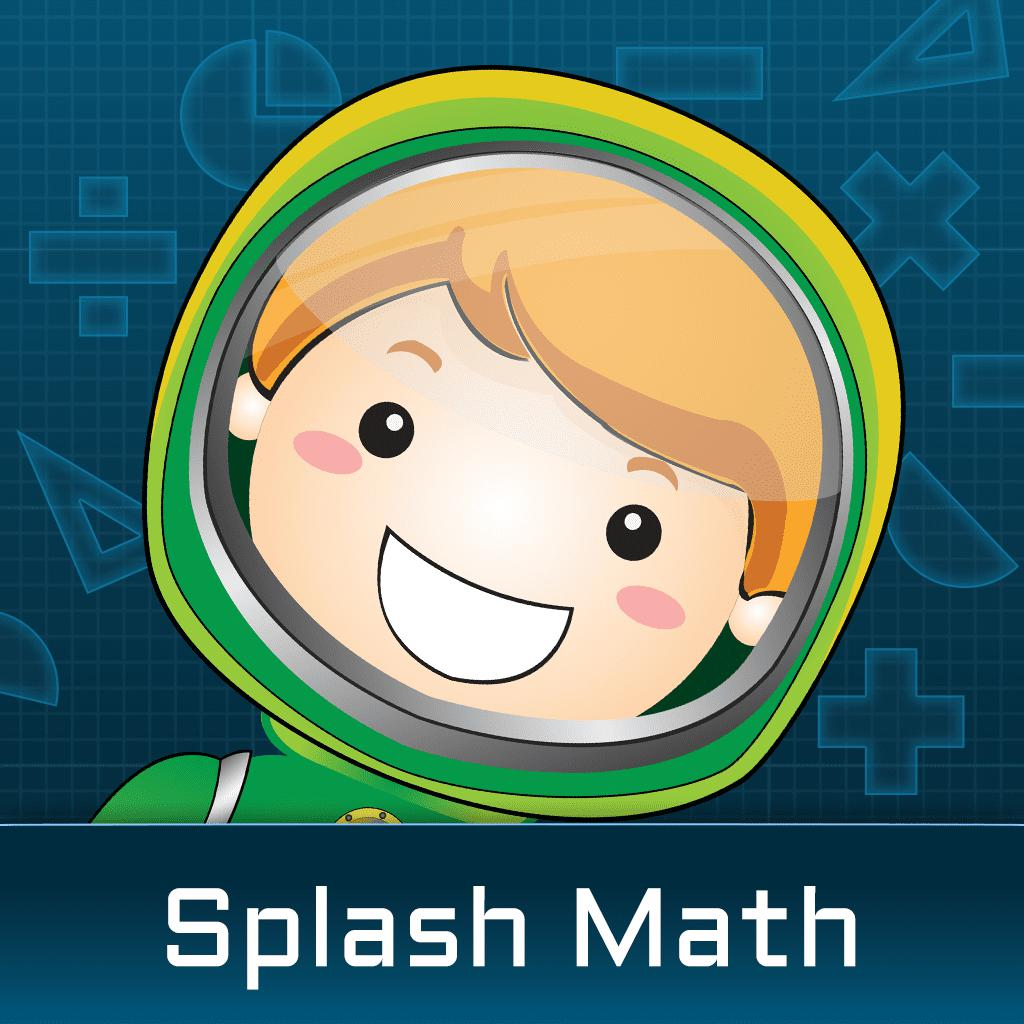Math Games for 5th Grade Kids-Learning Decimals & Fractions 4 738

"multiplication kids: math game" has 190 related apps. Top 5 apps are Multiplication Kids: Math Game, Monster Math : Kids Fun Games, Math Brain Booster Games, Cool Times Tables Flash Cards, and Math Games for 5th Grade Kids.

• timetable games  Related Apps & App Analytics
 Apps Average Star RatingsMultiplication Kids: Math Game-Multiplication Games for Kids 4 599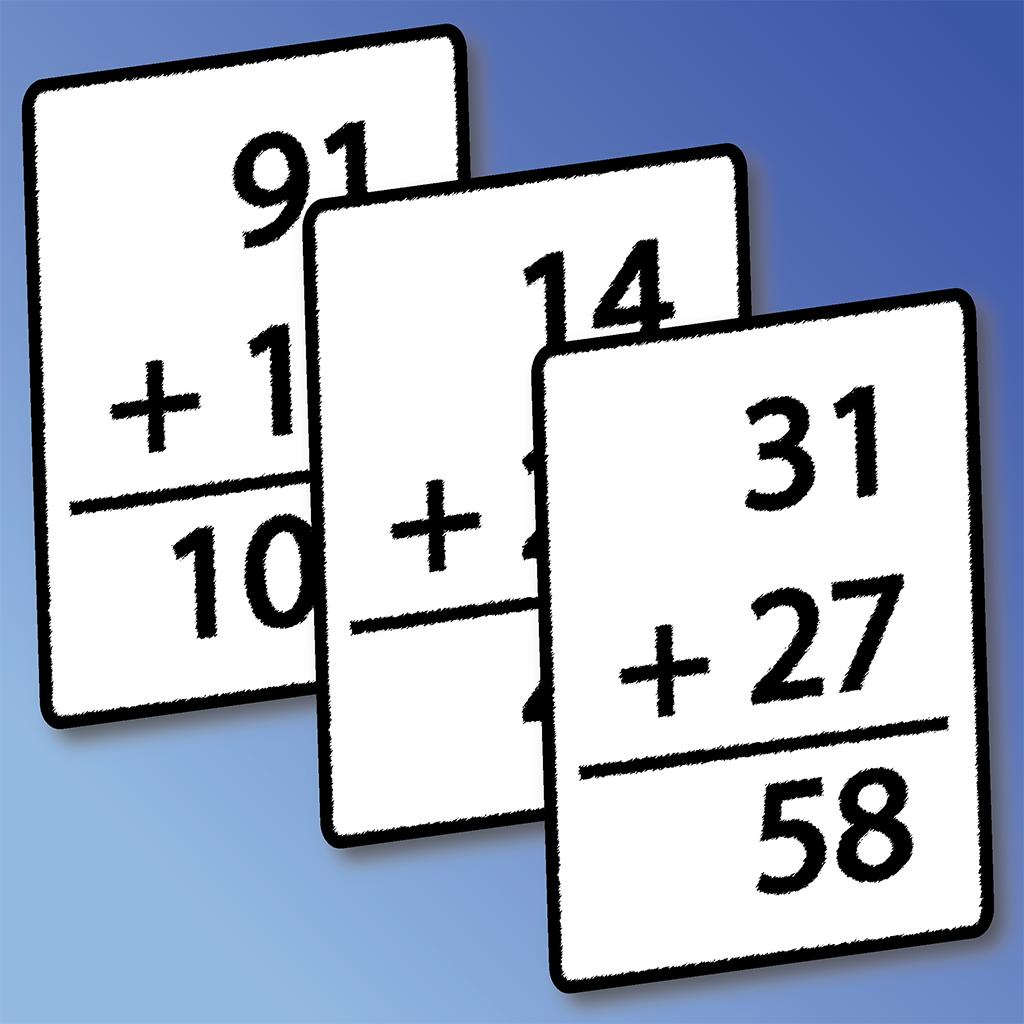Mental Math Cards Games & Tips-Challenge, Facts, & Practice 4 10652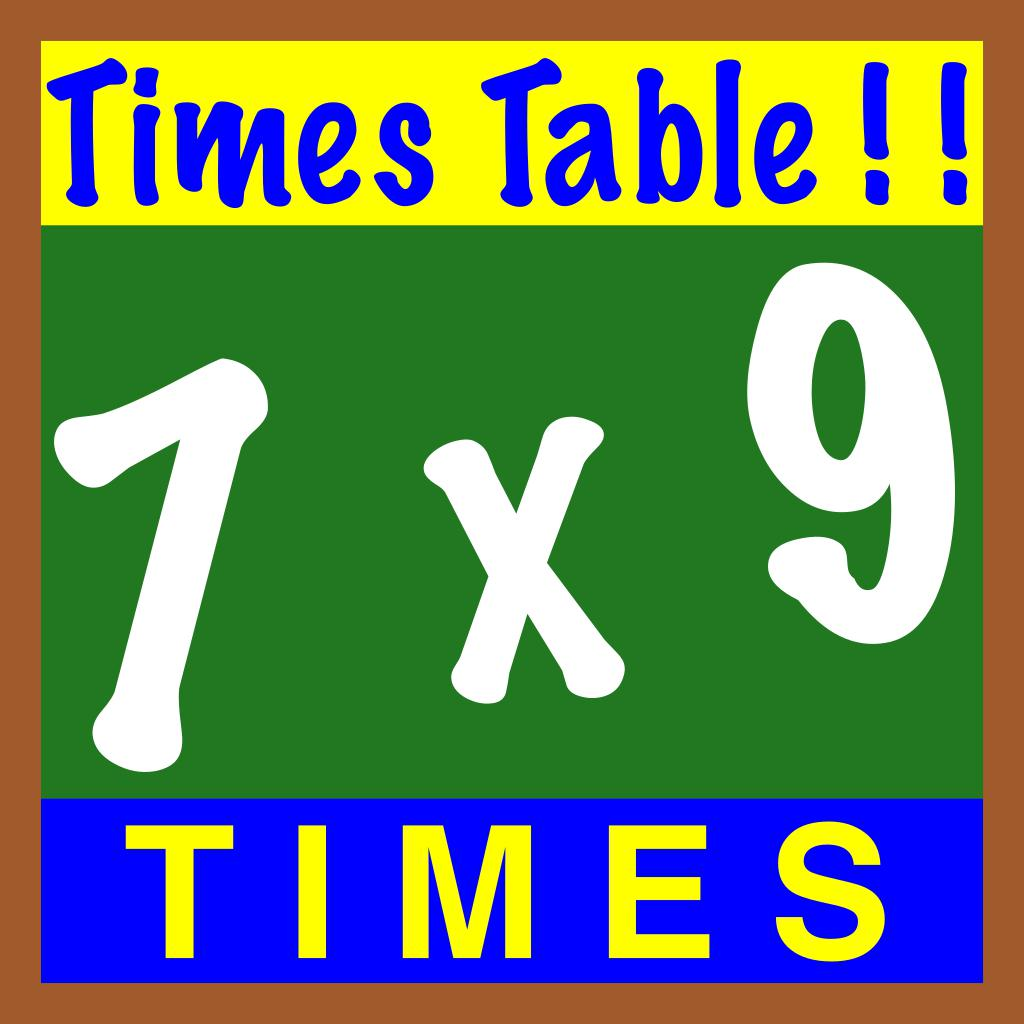Times Table ! ! 4 141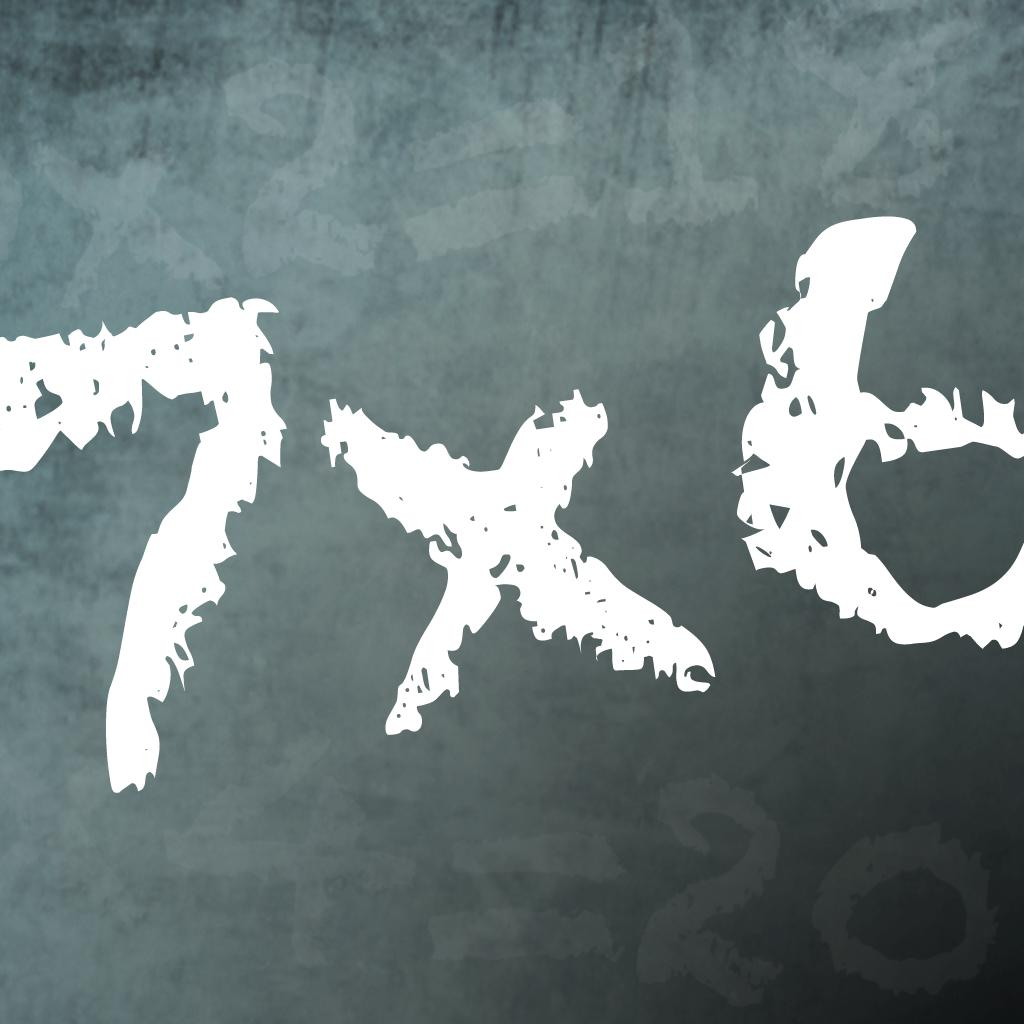Times Tables Quiz!-Multiplication Trainer 4 678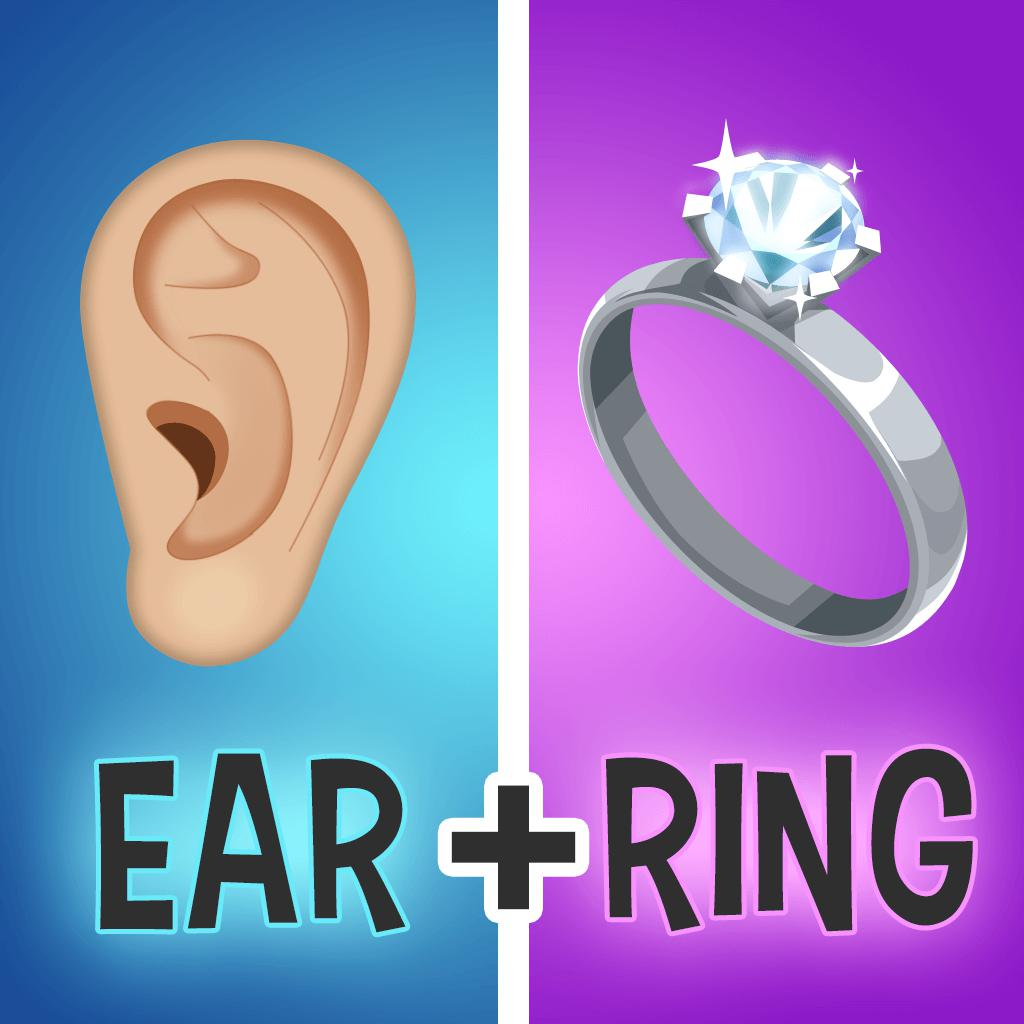Pictoword: Fun Word Quiz Games-Top Offline Picture Guess App 4 81924

223 apps are ranking for "timetable games" in app store. Top 5 apps are Multiplication Kids: Math Game, Mental Math Cards Games & Tips, Times Table ! !, Times Tables Quiz!, and Pictoword: Fun Word Quiz Games.

As you can see, Multiplication Kids: Math Game, Multiplication Kids: Math Game, and Big Math Flash Cards are ranking for multiplication games, also, Multiplication Kids: Math Game, Multiplication Kids: Math Game, Multiply: Disciples Making Disciples and Multiplication: Times Tables are all competing the keyword multiply.

Before promoting your apps in app store, you'd better perform app analytics for better ASO tactics, and ASOTools, free ASO tools will help you with App store keywords optimization.